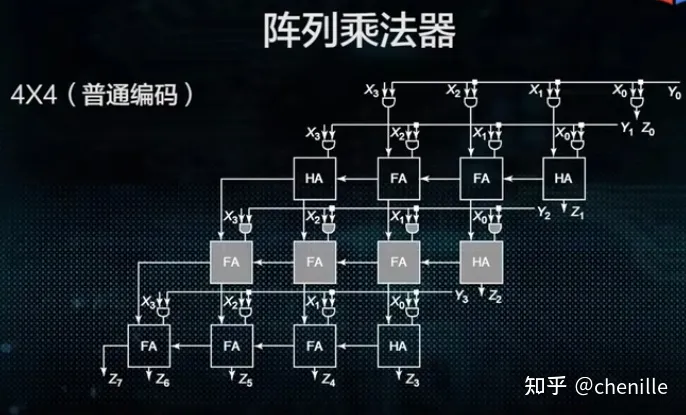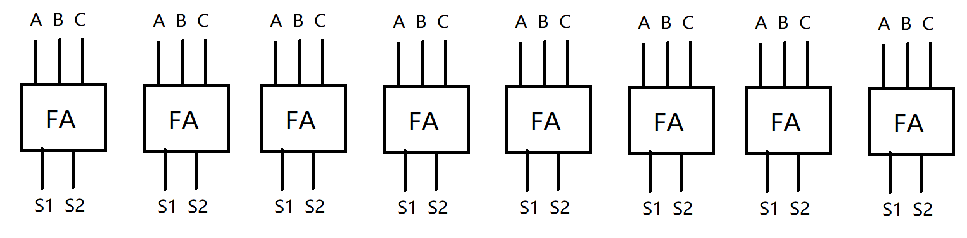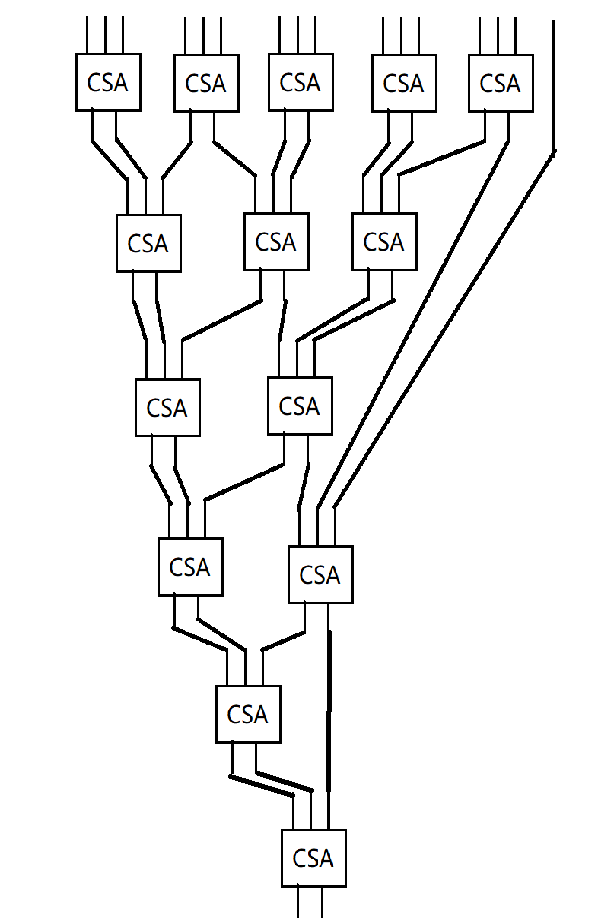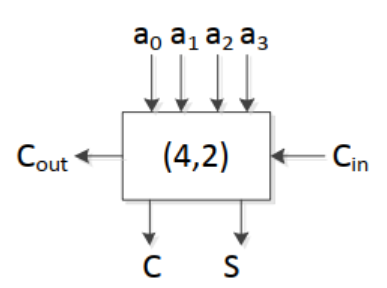# 阵列乘法器

$1011 * 0111$

（想一想10进制时，你是怎么做乘法的？）

$00000000$

$10110000$

$01011000$

$(1)00001000$ 相加

$10000100$ 移位

$(1)00110100$ 相加

$10011010$ 移位

$10011010$ 相加

$01001101$ 移位# 从小位宽到大位宽

$A[63:0] * B[63:0]$

$= (A[63:32]*2^{32} + A[31:0]) * (B[63:32]*2^{32} + B[31:0])$

$= (A[63:32] * B[63:32])*2^{64} + (A[63:32] * B[31:0])*2^{32} + (A[31:0] * B[63:32])*2^{32} + (A[31:0] * A[31:0])$

$= (A[63:32] * B[63:32]) << 64 + (A[63:32] * B[31:0]) << 32 + (A[31:0] * B[63:32]) << 32 + (A[31:0] * A[31:0])$

（32位的乘法器相信你能用同样的思路很快写出来）

# bonus: wallance树 和 CSA

## CSA：进位保留加法器## wallance树（孩子用触控板画的，别骂了）# bonus: 布斯乘法器

$y= -2^{n-1}y_{n-1} + \sum_{i=n-2}^{0}2^{i}y_{i}$

$y= -2^{n-1}y_{n-1} + \sum_{i=n-2}^{0}2^{i}y_{i} + y_{-1} \text{ , where } y_{-1} = 0$

$y= -2^{n-1}y_{n-1} + 2^{n-2}y_{n-2} + 2^{n-3}y_{n-3} + \dots + 2^{2}y_{2} + 2^{1}y_{1} + 2^{0}y_{0} + y_{-1} $\$= -2^{n-1}y_{n-1} + 2^{n-2}y_{n-2} \red{+ 2^{n-2}y_{n-2} - 2^{n-2}y_{n-2}} + 2^{n-3}y_{n-3} \red{+ 2^{n-3}y_{n-3} - 2^{n-3}y_{n-3}} + \dots + 2^{1}y_{1} \red{+ 2^{1}y_{1} - 2^{1}y_{1}} + 2^{0}y_{0} \red{+ 2^{0}y_{0} - 2^{0}y_{0}} + y_{-1} $\$ = -2^{n-1}y_{n-1} + \blue{2^{n-1}y_{n-2}} - \red{2^{n-2}y_{n-2}} + \blue{2^{n-2}y_{n-3}} - \red{2^{n-3}y_{n-3}} + \dots + \blue{2^{2}y_{1}} - \red{2^{1}y_{1}} + \blue{2^{1}y_{0}} - \red{2^{0}y_{0}} + y_{-1} $\$ = 2^{n-1}\blue{(-y_{n-1} + y_{n-2})} + 2^{n-2}\blue{(-y_{n-2} + y_{n-3})} + \dots + 2^{2}\blue{(-y_{2} + y_{1})} + 2^{1}\blue{(-y_{1}+y_{0})} + 2^{0}\blue{(-y_{0} + y_{-1})}$

$y = \red{-2}*2^{n-2}y_{n-1} + \red{2}2^{n-2}y_{n-2} + \red{22^{n-4}}y_{n-3} - 2^{n-3}y_{n-3} + \dots + \red{2}2^{1}y_{1} - \red{22^{0}}y_{1} + 2^{0}y_{0} + y_{-1}$

$y = -\red{22^{n-2}}y_{n-1} + \red{2^{n-2}}y_{n-2} + \red{2^{n-2}}y_{n-3} - \red{22^{n-4}}y_{n-3} + \red{2^{n-4}}y_{n-4} + \red{2^{n-4}}y_{n-5} + \dots + -22^{2}y_{3} + 2^{2}y_{2} + 2^{2}y_{1} - 22^{0}y_{1} + 2^{0}y_{0} + 2^{0}y_{-1} $\$y = 2^{n-2}(-2y_{n-1} + y_{n-2} + y_{n-3}) + 2^{n-4}(-2y_{n-3} + y_{n-4} + y_{n-5}) + \dots + 2^{2}(-2y_{3} + y_{2} + y_{1}) + 2^{0}(-2y_{1} + y_{0} + y_{-1})$

$n-2,n-4,n-6 \dots$

$x_{2i+1}$ $x_{2i}$ $x_{2i-1}$ $PP_{i}$
0 0 0 0
0 0 1 Y
0 1 0 Y
0 1 1 Y<<1
1 0 0 -Y<<1
1 0 1 -Y
1 1 0 -Y
1 1 1 0

# 那除法呢？

$(a * b) \equiv 1 \mod 2^{64}$

$a * b^{-1} \equiv a/b \mod 2^{64}$